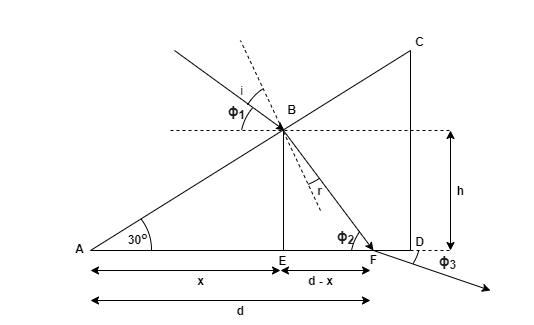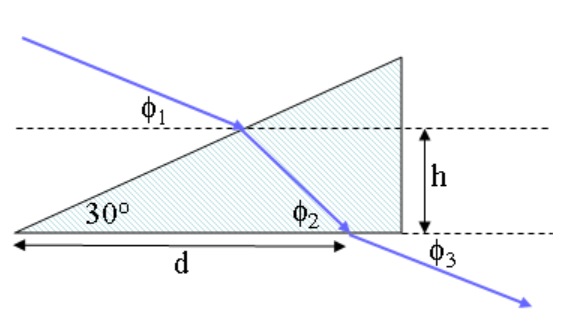# Problem: Red light is incident in air on a 30- 60- 90(degree) prism as shown. The incident beam is directed at an angle of ϕ1 = 41.3° and ϕ2 = 46.64° with respect to the horizontal and enters the prism at a height h = 17 cm above the base. The beam leaves the prism to the air at a distance d = 45.5 along the base as shown.What is n, the index of refraction of the prism for red light?

###### FREE Expert Solution

Snell's law:

$\overline{){{\mathbf{\eta }}}_{{\mathbf{1}}}{\mathbf{s}}{\mathbf{i}}{\mathbf{n}}{{\mathbf{\theta }}}_{{\mathbf{1}}}{\mathbf{=}}{{\mathbf{\eta }}}_{{\mathbf{2}}}{\mathbf{s}}{\mathbf{i}}{\mathbf{n}}{{\mathbf{\theta }}}_{{\mathbf{2}}}}$i = 90 - 30 - ϕ1 = 90 - 30 - 41.3 = 18.7°

From triangle ABC:###### Problem Details

Red light is incident in air on a 30- 60- 90(degree) prism as shown. The incident beam is directed at an angle of ϕ1 = 41.3° and ϕ2 = 46.64° with respect to the horizontal and enters the prism at a height h = 17 cm above the base. The beam leaves the prism to the air at a distance d = 45.5 along the base as shown.What is n, the index of refraction of the prism for red light?